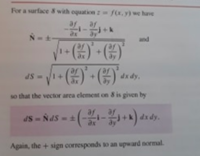# Evaluating integral for unknown C using Stokes' theorem

#### veggisheggis

##### New member
(translating from norwegian)
C is the intersection between the sylinder 7x2+y2=4, and a smooth(?) function f:R2→R, meaning the surface z=f(x,y).
The curve is oriented counter-clockwise viewed from above. Evaluate the integral:

∮C−y3dx+7x3dy−3z3dz.

This means: even though we don't know the curve C, it's possible to evaluate the integral. Find a surface(/area(?)) limited by C and use Stokes' theorem. This formula might help:(in the pink box)

#### veggisheggis

##### New member
C(−y3)dx+(7x3)dy(−3z3)dz